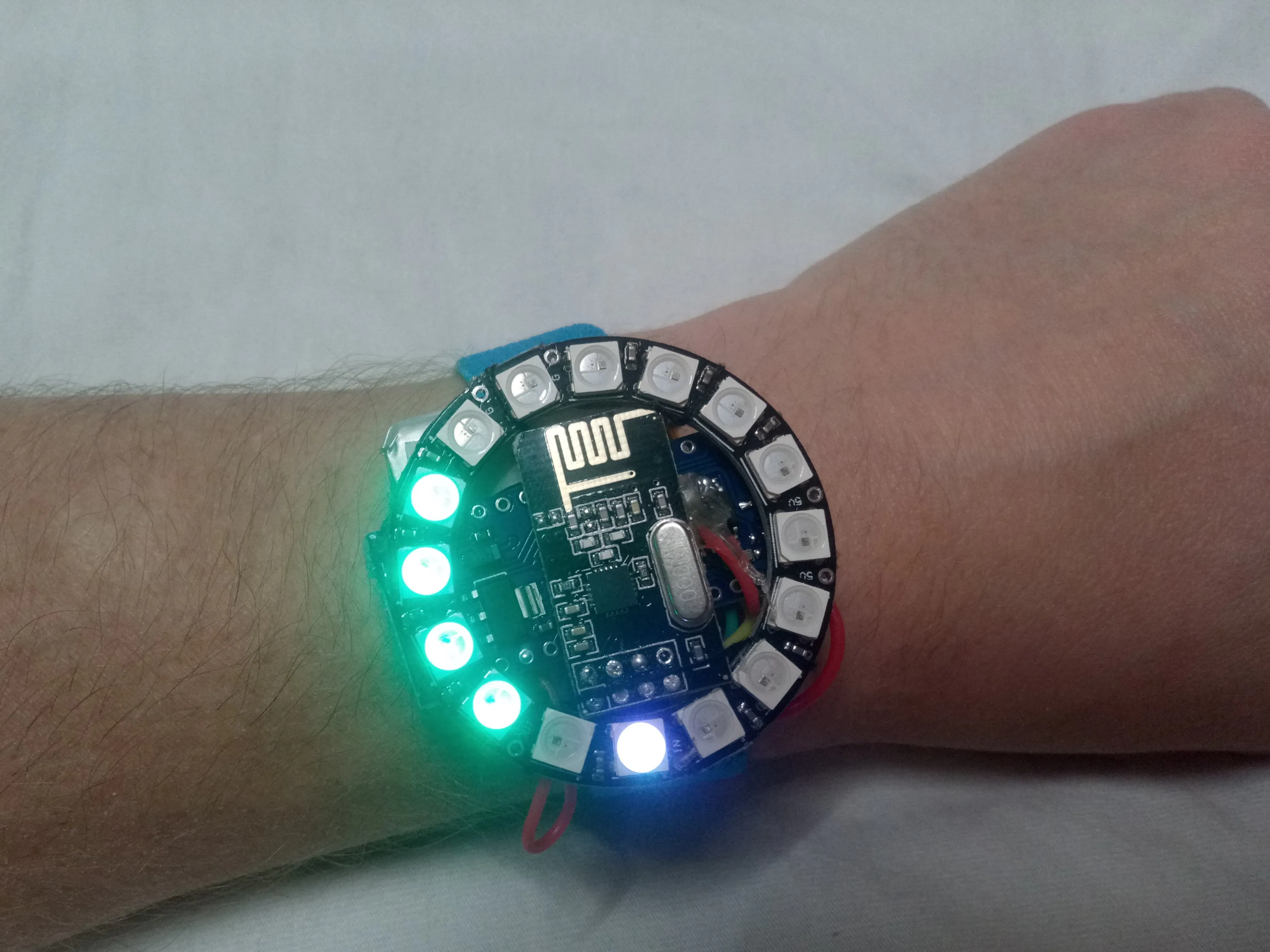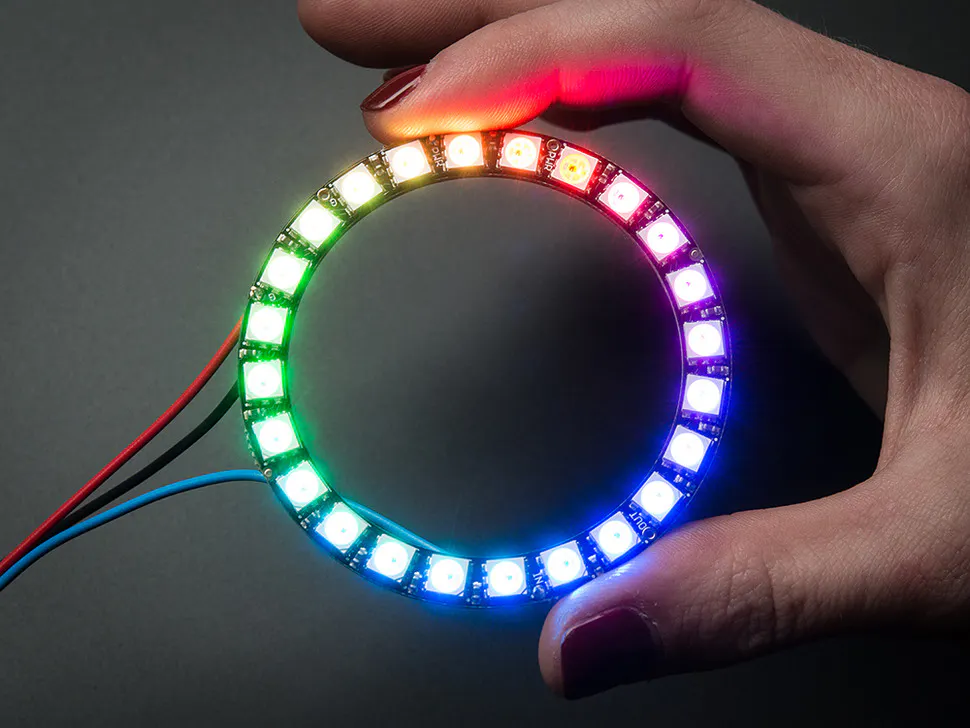# Heart Rate Monitor (Wearable and Wireless Using ECG)

A rather convenient device to monitor heart rate while running.

IntermediateFull instructions provided6 hours19,507## Things used in this project

### Hardware components

 uECG device For actually measuring BPM. It can send data via nRF24-compatible protocol
×1Arduino Nano R3
×1
 nRF24 Module (Generic) Any module would work here. Required to receive BPM data from uECG
×1Adafruit NeoPixel Ring: WS2812 5050 RGB LED
×1
 LiPo battery Any battery with 200+ mAh capacity
×1

### Hand tools and fabrication machinesSoldering iron (generic)

## Code

### bpm_watch.ino

Arduino
```#include <Adafruit_NeoPixel.h>
#ifdef __AVR__
#include <avr/power.h>
#endif

#include <SPI.h>
#include <RF24.h>
#include <RF24_config.h>
#include <nRF24L01.h>

int rf_cen = 9; //nRF24 chip enable pin
int rf_cs = 8; //nRF24 CS pin

RF24 rf(rf_cen, rf_cs);
//pipe address - hardcoded on uECG side
uint8_t pipe_rx = {0x0E, 0xE6, 0x0D, 0xA7, 0, 0, 0, 0};

// Which pin on the Arduino is connected to the NeoPixels?
#define PIN            5
// How many NeoPixels are attached to the Arduino?
#define NUMPIXELS      16

uint8_t  swapbits(uint8_t a){ //uECG pipe address uses swapped bits order
// reverse the bit order in a single byte
uint8_t v = 0;
if(a & 0x80) v |= 0x01;
if(a & 0x40) v |= 0x02;
if(a & 0x20) v |= 0x04;
if(a & 0x10) v |= 0x08;
if(a & 0x08) v |= 0x10;
if(a & 0x04) v |= 0x20;
if(a & 0x02) v |= 0x40;
if(a & 0x01) v |= 0x80;
return v;
}

void setup() {
pixels.begin(); // This initializes the NeoPixel library.

for(int i=0;i<NUMPIXELS;i++){
pixels.setPixelColor(i, pixels.Color(1,1,1));
}
pixels.show();

//nRF24 requires relatively slow SPI, probably would work at 2MHz too
SPI.begin();
SPI.setBitOrder(MSBFIRST);
SPI.beginTransaction(SPISettings(1000000, MSBFIRST, SPI_MODE0));

for(int x = 0; x < 8; x++) //nRF24 and uECG have different bit order for pipe address
pipe_rx[x] = swapbits(pipe_rx[x]);

rf.begin();
rf.setDataRate(RF24_1MBPS);
rf.setChannel(22);
rf.setRetries(0, 0);
rf.setAutoAck(0);
rf.setCRCLength(RF24_CRC_DISABLED);
rf.disableCRC();
rf.startListening(); //listen for uECG data
//Note that uECG should be switched into raw data mode (via long button press)
//in order to send compatible packets, by default it sends data in BLE mode
//which cannot be received by nRF24
}

long last_pix_upd = 0;
byte in_pack;
int rf_bpm = 0;
int bpm_hist; //since we disabled CRC, need to filter incoming data

void loop()
{
if(rf.available())
{
int bb = in_pack; //BPM is located at the 5th byte of the packet
//detailed packet structure is in uECG docs

//since we have no CRC for compatibility reasons, we need to filter
//incoming data, radio channel could be noisy. We compare last 5
//received BPM values and use one only if all 5 were the same.
//Since uECG sends about 100 packets per second, it won't cause
//any noticeable delay in displaying data
for(int n = 0; n < 5-1; n++) //shift bpm history array by 1
bpm_hist[n] = bpm_hist[n+1];
bpm_hist = bb; //add new bpm value
for(int n = 0; n < 5; n++) //check if all are equal
if(bpm_hist[n] != bb) bb = -1;
if(bb > 0) //if yes - store it as new received BPM
rf_bpm = bb;
}
long ms = millis();
if(ms - last_pix_upd > 10) //don't update pixels too often
{
int r, g, b;
last_pix_upd = ms;
int bpm = rf_bpm;
int max_bright = 160; //value of maximum brightness, max 255. But you don't always want it at max :)
float dd = 25; //change in BPM between color tones (blue->green->yellow->pink->red)
float t1 = 90, t2, t3, t4; //t1 - "base" BPM, lower than t1 would be blue
t2 = t1 + dd;
t3 = t2 + dd;
t4 = t3 + dd;
//code for changing color depending in which t1...t4 range we are now
if(bpm < t1){ r = 0; g = 0; b = max_bright; }
else if(bpm < t2) { r = 0; g = max_bright * (bpm-t1)/dd; b = max_bright - g; }
else if(bpm < t3) { r = max_bright * (bpm-t2)/dd; g = max_bright - r; b = r/4; }
else if(bpm < t4) { r = max_bright; g = 0; b = max_bright/2 - max_bright * (bpm-t3)/(2*dd); }
else {r = max_bright; g = 0; b = 0; }
int on_pixels = (bpm-80)/8; //since it's intended for running, I'm not
//showing anything less than 80 BPM, this way it's more sensitive in
for(int i=0;i<NUMPIXELS;i++)
{
//pixels are set from last to first for no particular reason, would
//work just as fine if set from first to last
if(i < on_pixels) pixels.setPixelColor(NUMPIXELS-i-1, pixels.Color(r,g,b));
else pixels.setPixelColor(NUMPIXELS-i-1, pixels.Color(0,0,0)); //turn off all other LEDs
}
pixels.show();
}
}
```

## Credits

### Dmitry Dziuba

3 projects • 34 followers
I'm an electronics engineer with background in AI and physics